# Related Videos

## Factor the expression $121+198x^6+81x^{12}$

Go!
1
2
3
4
5
6
7
8
9
0
a
b
c
d
f
g
m
n
u
v
w
x
y
z
.
(◻)
+
-
×
◻/◻
/
÷
2

e
π
ln
log
log
lim
d/dx
Dx
|◻|
=
>
<
>=
<=
sin
cos
tan
cot
sec
csc

asin
acos
atan
acot
asec
acsc

sinh
cosh
tanh
coth
sech
csch

asinh
acosh
atanh
acoth
asech
acsch

### Videos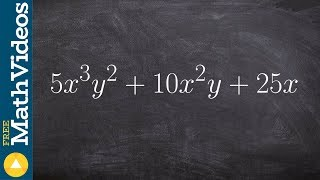### Algebra 1 - Factoring three terms with distributive property 5x^3 y^2 + 10x^2y + 25x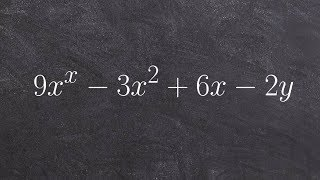### Tutorial - Factor an expression by using grouping, 9x^2 - 3xy + 6x - 2y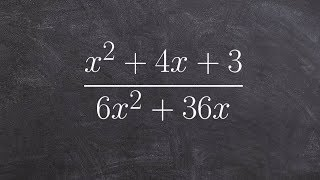### Simplify a rational expression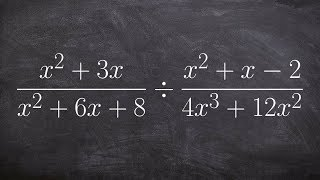### Tutorial - Simplifying by factoring to mulitply two rational expressions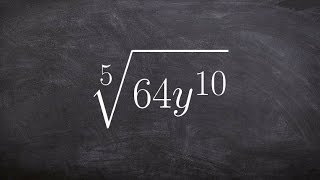### Simplify the 5th root of an expression using a factor tree and product prop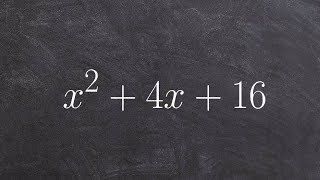### How do you factor a perfect square trinomial

$factor\left(121+198x^6+81x^{12}\right)$